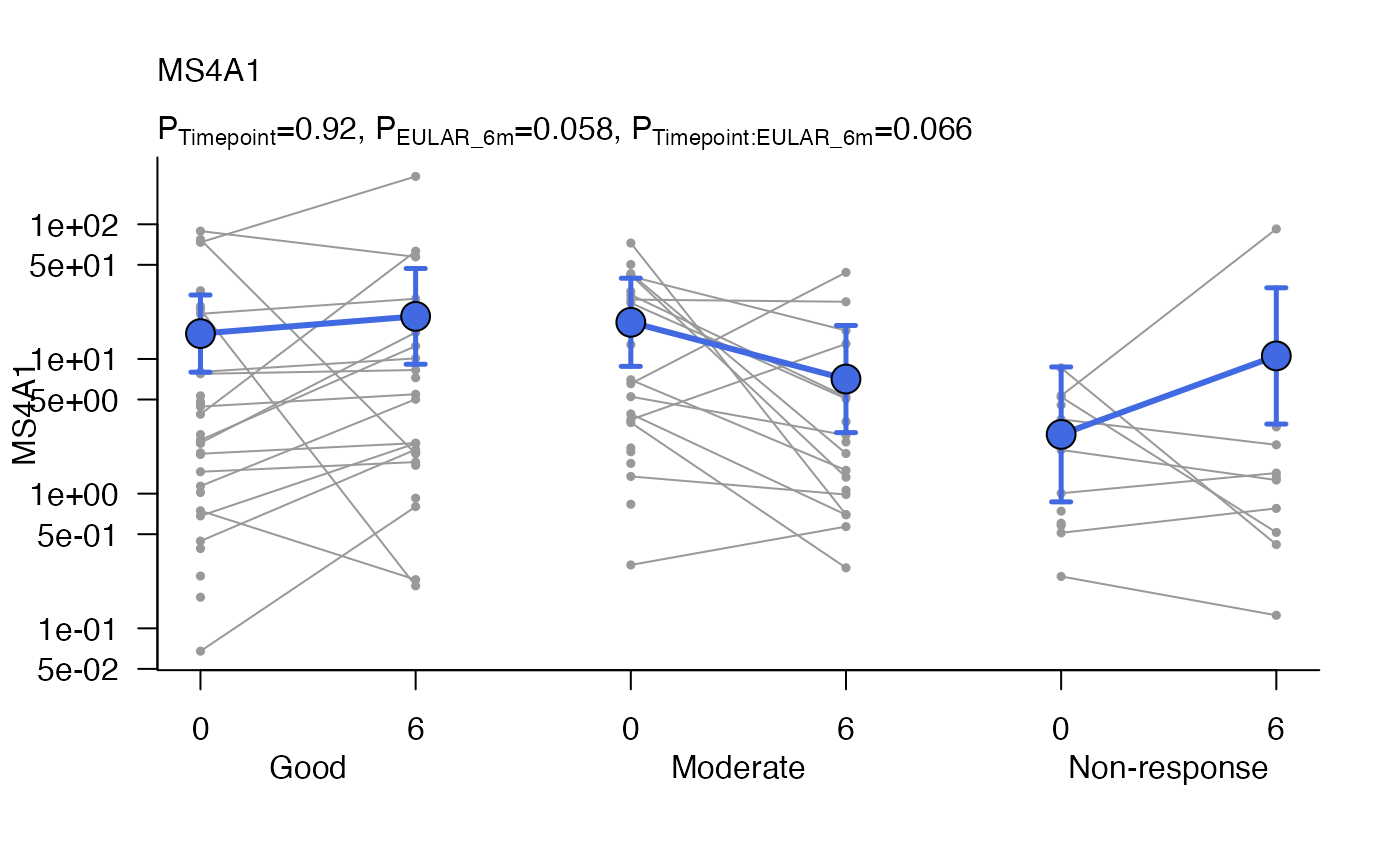Plot to show differences between groups over time using base graphics.

## Usage

modelPlot(
object,
geneName = NULL,
x1var = NULL,
x2var = NULL,
x2shift = NULL,
xlab = NA,
ylab = geneName,
plab = NULL,
title = geneName,
logTransform = is(object, "GlmmSeq"),
shapes = 21,
colours = "grey60",
lineColours = "grey60",
markerSize = 0.5,
fontSize = NULL,
alpha = 0.7,
modelSize = 2,
modelColours = "royalblue",
modelLineSize = 1,
modelLineColours = modelColours,
errorBarLwd = 2.5,
errorBarLength = 0.05,
...
)

## Arguments

object

A glmmSeq/lmmSeq object created by glmmSeq::glmmSeq() or glmmSeq::lmmSeq()

geneName

The gene/row name to be plotted

x1var

The name of the first (inner) x parameter, typically 'time'. This is anticipated to have different values when matched by ID.

x2var

The name of an optional second (outer) x parameter, which should be a factor.

x2shift

Amount to shift along x axis for each level of x2var. By default the function will arrange each level of x2var side by side. Lower values of x2shift or x2shift = 0 can be used to overlap plots similar to 'dodge' or stagger them.

xlab

Title for the x axis

ylab

Title for the y axis

plab

Optional character vector of labels for p-values. These must align with column names in object@stats\$pvals.

title

Plot title. If NULL gene name is used

logTransform

Whether to perform a log10 transform on the y axis

shapes

The marker shapes (default=19)

colours

The marker colours (default='red') as vector or named vector

lineColours

The line colours (default='grey60') as vector or named vector

markerSize

Size of markers (default=2)

fontSize

Plot font size

alpha

Line and marker opacity (default=0.7)

Whether to add the fit model with markers (default=TRUE)

Whether to add underlying data points (default=TRUE)

modelSize

Size of model points (default=2)

modelColours

Colour of model fit markers (default="black") as vector or named vector

modelLineSize

Size of model points (default=1) as vector or named vector

modelLineColours

Colour of model fit lines.

errorBarLwd

Line width of error bars

errorBarLength

...

Other parameters to pass to graphics::plot()

## Value

Returns a paired plot for matched samples

## Examples

data(PEAC_minimal_load)

disp <- apply(tpm, 1, function(x){
(var(x, na.rm=TRUE)-mean(x, na.rm=TRUE))/(mean(x, na.rm=TRUE)**2)
})

MS4A1glmm <- glmmSeq(~ Timepoint * EULAR_6m + (1 | PATID),
countdata = tpm[1:2, ],